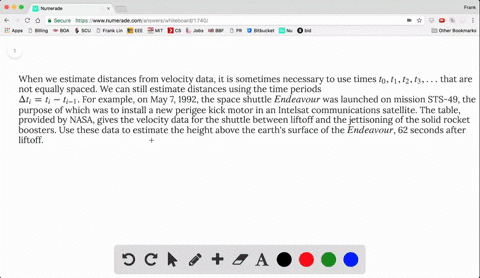### When we estimate distances from velocity data, it…

01:08
FL
Problem 15

Oil leaked from a tank at a rate of $r(t)$ liters per hour. The rate decreased as time passed and values of the rate at two-hour time intervals are shown in the table. Find lower and upper estimates for the total amount of oil that leaked out.

$L_{5}=70$liters$, R_{5}=63.2$liters

## Discussion

You must be signed in to discuss.

## Video Transcript

here, give you the oil leaking rate and ask you to find the finds. Ah, it's a total amount of oil that leaks out so again the time into those two hours. And what is the lower estimate? The lower estimate for each in time interval we use the smallest possible e great Assauer estimate so far is that from zero to two, we pick seven point six against the table. It's on your textbook. From two to four. We pick a six point eight six point two from six to eight. We put five point seven from eight to ten. We pick five points. Three a simile for up her ah each interval pictures the largest leaking rate for for our estimate and each time into those two hour. By the way, the unit's leader here and so the first one should be a point seven. The second one seven point six six point eight six point two five point seven